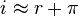Tags
#finance #has-images #inflation
Question

Letting r denote the real interest rate, i denote the nominal interest rate, and let π denote the [...], the Fisher equation is:This is a linear approximation,

inflation rate

Tags
#finance #has-images #inflation
Question

Letting r denote the real interest rate, i denote the nominal interest rate, and let π denote the [...], the Fisher equation is:This is a linear approximation,

?

Tags
#finance #has-images #inflation
Question

Letting r denote the real interest rate, i denote the nominal interest rate, and let π denote the [...], the Fisher equation is:This is a linear approximation,

inflation rate
If you want to change selection, open original toplevel document below and click on "Move attachment"

#### Parent (intermediate) annotation

Open it
Letting r denote the real interest rate, i denote the nominal interest rate, and let π denote the inflation rate, the Fisher equation is: This is a linear approximation,

#### Original toplevel document

Fisher equation - Wikipedia, the free encyclopedia
his works on the theory of interest. In finance, the Fisher equation is primarily used in YTM calculations of bonds or IRR calculations of investments. In economics, this equation is used to predict nominal and real interest rate behavior. <span>Letting r denote the real interest rate, i denote the nominal interest rate, and let π denote the inflation rate, the Fisher equation is: This is a linear approximation, but as here, it is often written as an equality: The Fisher equation can be used in either ex-ante (before) or ex-post (after) analysis. Ex-post, it can be used to describe the real p

#### Summary

status measured difficulty not learned 37% [default] 0

No repetitions